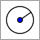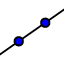# Constructions: Square Inscribed in a Circle APS

## Explore the geometric properties of a square inscribed in a circle.

Summarize the properties of squares, circles, diameters, chords,and how they would relate if the square is inscribed in a circle, before you start your actual construction.  Hint: If you are not familiar with the steps necessary for inscribing square in a circle construction, you might want to explore the applet below. Just use the buttons of the Navigation Bar in order to replay the construction steps.

Construct a square inscribed within a circle by following the construction steps provided below.

## Construction Steps:

 ﻿1. ﻿Construct a circle with center A through B. (circle c) 6.﻿ ﻿﻿Construct a circle with the center at point B and radius of f+.5. (circle e) ﻿2. ﻿Construct segment AB.﻿ (segment f) ﻿7. ﻿Construct the intersection points between circles d & e. (points D & E) ﻿3. ﻿﻿Construct line AB.﻿ (line g) ﻿8. ﻿﻿Construct line DE.﻿ (line h) ﻿4. ﻿﻿Construct the intersection point between line g and circle c. (point C) 9. ﻿Construct the intersection points between line h and circle c. (points G & F) ﻿5. ﻿﻿Construct a circle with the center at point C and radius of f+.5. (circle d) ﻿10. ﻿﻿Draw Quadrilateral BFCG. (poly1)